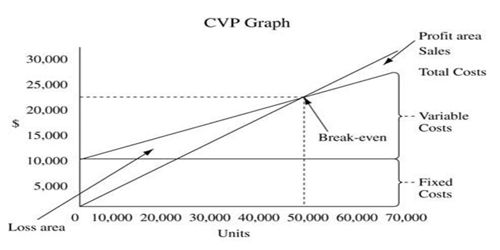Accounting

# Uses of CVP AnalysisCost-volume-profit (CVP) analysis is used to determine how changes in costs and volume affect a company’s operating income and net income. CPV analysis is a powerful tool that helps managers understands the relationships of cost volume and profit. It deals with how operating profit is affected by changes in variable costs, fixed costs, selling price per unit and the sales mix of two or more different products.

In performing this analysis, there are several assumptions made, including:

• Constant sales price;
• Constant variable cost per unit;
• Constant total fixed cost;
• Constant sales mix;
• Units sold equal units produced.

Further use of CVP

CVP can answer some basic questions like:

• What will happen to profit if we change the selling prices?
• How will changes in variable costs or fixed costs impact planned profits?